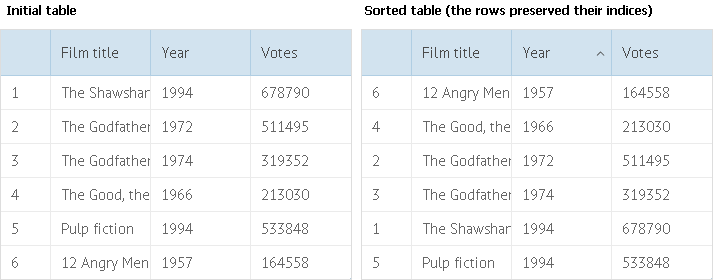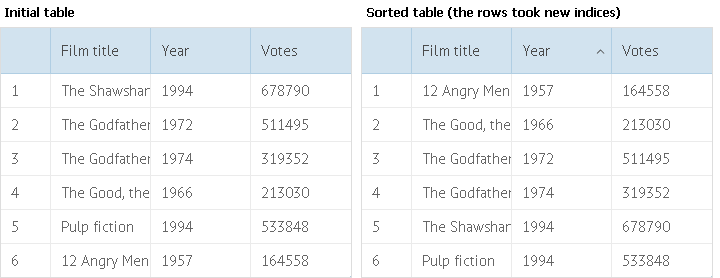# Creating Index Columns

You can create index columns in DataTable.

There are 2 types of index columns:

• Static (rows indexes aren't changed whether you move, delete rows etc.)
• Dynamic (rows indexes can vary depending on the current position of rows)

## Static Index Columns

To create a static index column in your datatable, use the following technique:Specifying static index column in DataTable

``````dtable = webix.ui({
view:"datatable",
columns:[
],
scheme:{
\$init:function(obj){ obj.index = this.count(); }
},
...
});``````

Related sample:  Index Column

Let's look more closely at the code above:

1. In the columns parameter we add a column to serve as the index entry.
2. Then, we add the \$init key to the scheme.

The code inside the \$init key runs when data are being loaded to the component initially and when data are being reloaded for new elements. The code is called for each data item, that's why we can use it as an iterator to assign indexes to rows.

## Dynamic Index Columns

To create a dynamic index column in your datatable, use the following technique:Specifying dynamic index column in DataTable

``````dtable = webix.ui({
view:"datatable",
id:"mytable",
columns:[
],
on:{
"data->onStoreUpdated":function(){
this.data.each(function(obj, i){
obj.index = i+1;
});
}
},
...
});``````

Related sample:  Index Column

Let's look more closely at the code above:

1. In the columns parameter we add a column to serve as the index entry.
2. With the on parameter we attach a handler to the onStoreLoad event.
"data->onStoreUpdated" is equal to \$\$('mytable').data.attachEvent('onStoreUpdated', function(){...})
3. The onStoreLoad event fires after data are changed in the data store.
4. The each method allows us to iterate through the collection of data items.